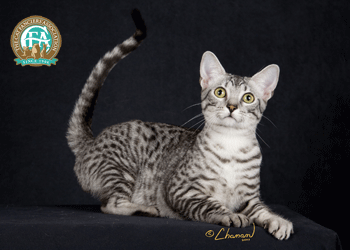# RuntimeError: number of dims don't match in permute

I ran into this error with notebook 08_pets_tutorial:

``````img = resized_image(items)
---------------------------------------------------------------------------
RuntimeError                              Traceback (most recent call last)
<ipython-input-58-d76e911b214c> in <module>
----> 1 img = resized_image(items)

<ipython-input-13-4b2ef1648cbb> in resized_image(fn, sz)
2     x = Image.open(fn).resize((sz,sz))
3     # Convert image to tensor for modeling
----> 4     return tensor(array(x)).permute(2,0,1).float()/255.

RuntimeError: number of dims don't match in permute
``````

Looks like this is caused by the fact that for some images (there are 9 of them) in `URLs.PETS` dataset, `array(Image.open(fn))` returns an array with `ndim=2` instead of 3. More details in this notebook: https://gist.github.com/yang-zhang/bea20a7e9791c341507e934e53a107fe

What’s the best way to handle these images? Thank you.

There is probably a `convert('RGB')` missing after opening the image with PIL.

2 Likes

I’m looking at this too. This is the image of one of the 2 dim images:Which seems really strange that it could have color. Here is the file I’m seeing this on:

PosixPath(’/home/kbird/.fastai/data/oxford-iiit-pet/images/Egyptian_Mau_139.jpg’)

And the `.shape` of the image is: (250, 350) which I’m not sure how it could have color if it doesn’t have that RGB dimension.

After reading more about this and debugging it, I think the reason this is a weird one is that it is of type `PIL.GifImagePlugin.GifImageFile`. Now I’m researching how that works, but it looks like PIL may store the colors in some other format for GIF Images

Expanding on this:

The problem is that GIF Images store everything in a single channel (somehow). To get resize to work correctly here, you need to change it to have a `.convert('RGB')` so resize will look like this:

``````def resized_image(fn:Path, sz=128):
x = Image.open(fn).resize((sz,sz)).convert("RGB")
# Convert image to tensor for modeling
return tensor(array(x)).permute(2,0,1).float()/255.
``````

The other way to do it would be by figuring out if it’s a gif type, that would probably save some time doing the convert on every image. I haven’t been able to test this though because my type check doesn’t work like I would expect it to.

2 Likes

This conditional conversion of GIF images solves the problem:

``````def resized_image(fn:Path, sz=128):
x = Image.open(fn).resize((sz,sz))
x = x.convert('RGB') if x.format=='GIF' else x
# Convert image to tensor for modeling
return tensor(array(x)).permute(2,0,1).float()/255.
``````

@sgugger, does it worth a PR?

2 Likes

Yes, though I think you can do the convert every time, not just when it’s a GIF. The usual `PILImage.open` does that (unless you use `PILImageBW` which converts to ‘L’).